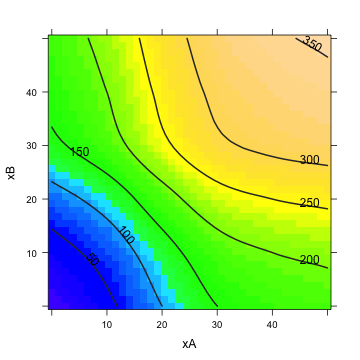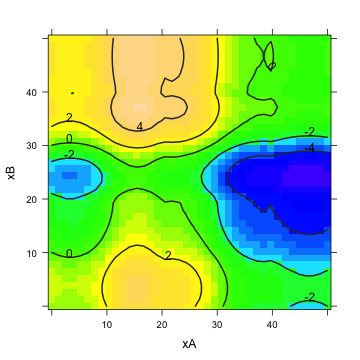# QALY 3

You are a member of a health-care oversight committee that allocates funds for investment in health-care facilities. The committee has two proposal before it and needs to decide how best to spend the available
$50M budget: • Proposal A: Expansion of the pediatric organ transplant unit. • Proposal B: Creation of a new rehabilitation center for traumatic injury patients. Experts have evaluated each proposal in terms of different cost options and the impact that each will have on health outcomes. Following a standard epidemiological method, the impact is estimated in terms of “Quality Adjusted Life-Years'' (QALY), a measure that combines the number of people affected, the number of years of prolonged life, and the improvement in quality of life for those treated. Here are the expert estimates: \begin{centering} QALY Impact of the Proposals \begin{tabular}{lrrrrrr} Expenditure & 0 & 10 & 20 & 30 & 40 & 50\\\hline Proposal A & 0 & 40 & 100 & 150 & 170 & 180\\ Proposal B & 0 & 30 & 80 & 140 & 160 & 175\\ \end{tabular} \end{centering}  Such estimates from experts should be taken with a grain of salt, but they are often the best information you have to inform a model. You can turn the expert's opinions into functions by using splines. In this case, there is good reason to think that output will increase monotonically with expenditure, so a monotonic spline is a good choice. You can construct the functions like this: expend = c(0, 10, 20, 30, 40, 50) A = c(0, 40, 100, 150, 170, 180) B = c(0, 30, 80, 140, 160, 175) dat = data.frame(expend = expend, A = A, B = B) fA = spliner(A ~ expend, data = dat, monotonic = TRUE) fB = spliner(B ~ expend, data = dat, monotonic = TRUE)  The problem is to find the best values for expenditures on Proposal A and B — call these expenditures $$x_A$$ and $$x_B$$ — given constraint that total expenditure is $$x_A + x_B = 50$$. A simple approach is to plot out the total benefit as a function of expenditures on each of A and B: plotFun(fA(xA) + fB(xB) ~ xA & xB, xA.lim = range(0, 50), xB.lim = range(0, 50))What's the largest number of QALYs that can be produced from combining the two proposals with a total budget of$50M? \SelectSetHoriz{240}{120,160,180,240,300,340,350,360}

What are the inputs $$x_A$$ and $$x_B$$ that produce this optimal outcome? Choose the closest answer:

• Input to A: $$x_A$$ \SelectSetHoriz{22}{0,6,12,22,34,41,50}
• Input to B: $$x_B$$ \SelectSetHoriz{28}{50,44,38,28,16,9,0}

Another way to look at the problem is to find the total QALY outcome as a function of the amount $$x_A$$, recognizing that once $$x_A$$ is set, the remaining money will go to option B, so $$x_B = 50-x_A$$.

Make a plot of total QALY outcome as a function of $$x_A$$. Which value of $$x_A$$ produces the best outcome? Zoom in on the graph around the maximum to find the location precisely.

\begin{AnswerText}

r
with50 = makeFun(fA(xA) + fB(50 - xA) ~ xA)
plotFun(with50(xA) ~ xA, xA.lim = range(0, 50))


![plot of chunk splineans](figure/splineans.png)

r
d50 = D(with50(xA) ~ xA)
findZeros(d50(xA) ~ xA, xA.lim = range(0, 50))



##  22.13




### Changing the Budget

A broader social question is whether the budget is being set at an appropriate level. One way to examine this is to look at how the QALY outcome changes as the budget changes.

1. What is the rate of change of $$f_A(x_A)$$ with respect to $$x_A$$? Of course, this depends on the value of $$x_A$$, so evaluate the derivative at the optimal expenditure.
2. What is the rate of change of $$f_B(x_B)$$ with respect to $$x_B$$? Again, evaluate this at the optimal expenditure (keeping in mind that the expenditure on B is $$50-x_A$$).
3. Using the chain rule, simplify the expression $$f_A(x_A) +f_B(50-x_A)$$ and show that, if you are at the optimal values of $$x_A$$ and $$x_B$$, it must be the case that $$\frac{\partial}{\partial x_A} f_A( x_A) = \frac{\partial}{\partial x_B} f_B (x_B)$$

This suggests another way to look at the optimum, plotting out the
difference between the derivatives to find inputs $$x_A$$ and $$x_B$$
where they are equal.

dfA = D(fA(xA) ~ xA)
dfB = D(fB(xB) ~ xB)
plotFun(dfA(xA) - dfB(xB) ~ xA & xB, xA.lim = range(0, 50), xB.lim = range(0,
50))There are many pairs of values $$(x_A, x_B)$$ on this graph where the two derivatives are equal. Find several. Then explain how the optimal value given the budget constraint $$x_A + x_B = 50$$ corresponds to just one of these.

\begin{AnswerText}
The optimal point is at the intersection of the constraint (shown in red) and the set of points where the two derivatives are equal.

r
plotFun(dfA(xA) - dfB(xB) ~ xA & xB, xA.lim = range(0, 50), xB.lim = range(0,
50))
plotFun(50 - xA ~ xA, add = TRUE, col = "red")




### Political Constraints: The Art of the Possible

The American Association of Allergy Activists (AAAA) has lobbied Congress to mandate that, of the 50 units of available funds, funding for A must be $$x_A \geq 40$$.

How much would this constraint reduce the overall output for the two interventions combined? (Remember, if you're spending, say, 45 on A, you can't spend more than the remaining 5 on B.)

\begin{MultipleChoice}
\wrong{Not at all.}
\wrong{It would actually {\bf increase} the output.}
\end{MultipleChoice}

\begin{AnswerText}
The best QALY value that satisfies both constraints $$x_A \geq 40$$ and
$$x_A + x_B = 50$$ is about 200 QALYs: a reduction of about 40 QALYs
from the case where there is no political constraint imposed.

r
overall = plotFun(fA(xA) + fB(xB) ~ xA & xB)
plotFun(xA >= 40 ~ xA & xB, xA.lim = range(0, 50), xB.lim = range(0,
50), add = TRUE, transparency = 0.2, lwd = 2)


![plot of chunk political](figure/political.png)




r
plotFun(50 - xA ~ xA, add = TRUE, col = "red", lwd = 2)